# Olympiad Test: Algebraic Expressions And Their Identities - 2

## 10 Questions MCQ Test Mathematical Olympiad Class 8 | Olympiad Test: Algebraic Expressions And Their Identities - 2

Description
Attempt Olympiad Test: Algebraic Expressions And Their Identities - 2 | 10 questions in 20 minutes | Mock test for Class 8 preparation | Free important questions MCQ to study Mathematical Olympiad Class 8 for Class 8 Exam | Download free PDF with solutions
QUESTION: 1

### What is the quotient if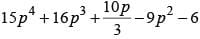is divided by 3p – 2 ?

Solution: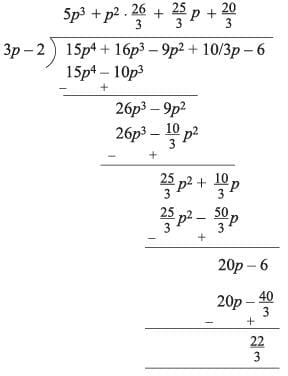QUESTION: 2

### What is the H.C.F. of 11abc3,  13a2 b2 c and 17ab3 c2 ?

Solution:

H.C.F. of 11abc3, 13a2b2c, 17ab3c2 = abc

QUESTION: 3

### ​If one of the factor of x 2 - y2+ 2yz - x2 is ( x + y-z) then what is the other factor?

Solution: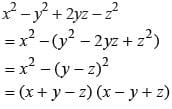QUESTION: 4

If one of the factor of x4 + x2 + 1 is (x2 + x + 1) then what is the other factor?

Solution: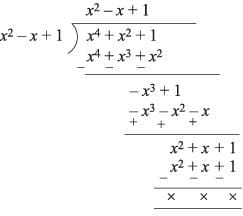QUESTION: 5

What are the factors of 11a2 + 54a + 63?

Solution: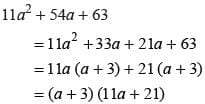QUESTION: 6

​What is the degree of the polynomial?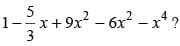Solution: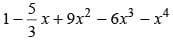Degree of the polynomial = Highest power of x = 4

QUESTION: 7

Find the remainder when x4 + 4x2 + 10 is divided by x2 - 2x + 4.

Solution: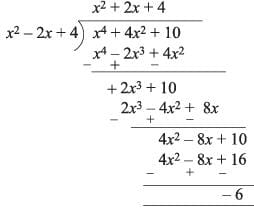QUESTION: 8

Which of the following is not a polynomial ?

Solution:

Clearly x 2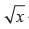− 2 =0 is not a polynomial.

QUESTION: 9

What is the product of the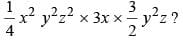Solution: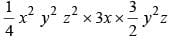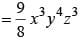QUESTION: 10

​If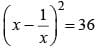then what is the value of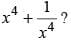Solution: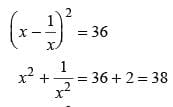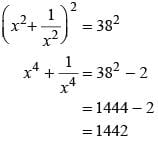Use Code STAYHOME200 and get INR 200 additional OFF Use Coupon Code